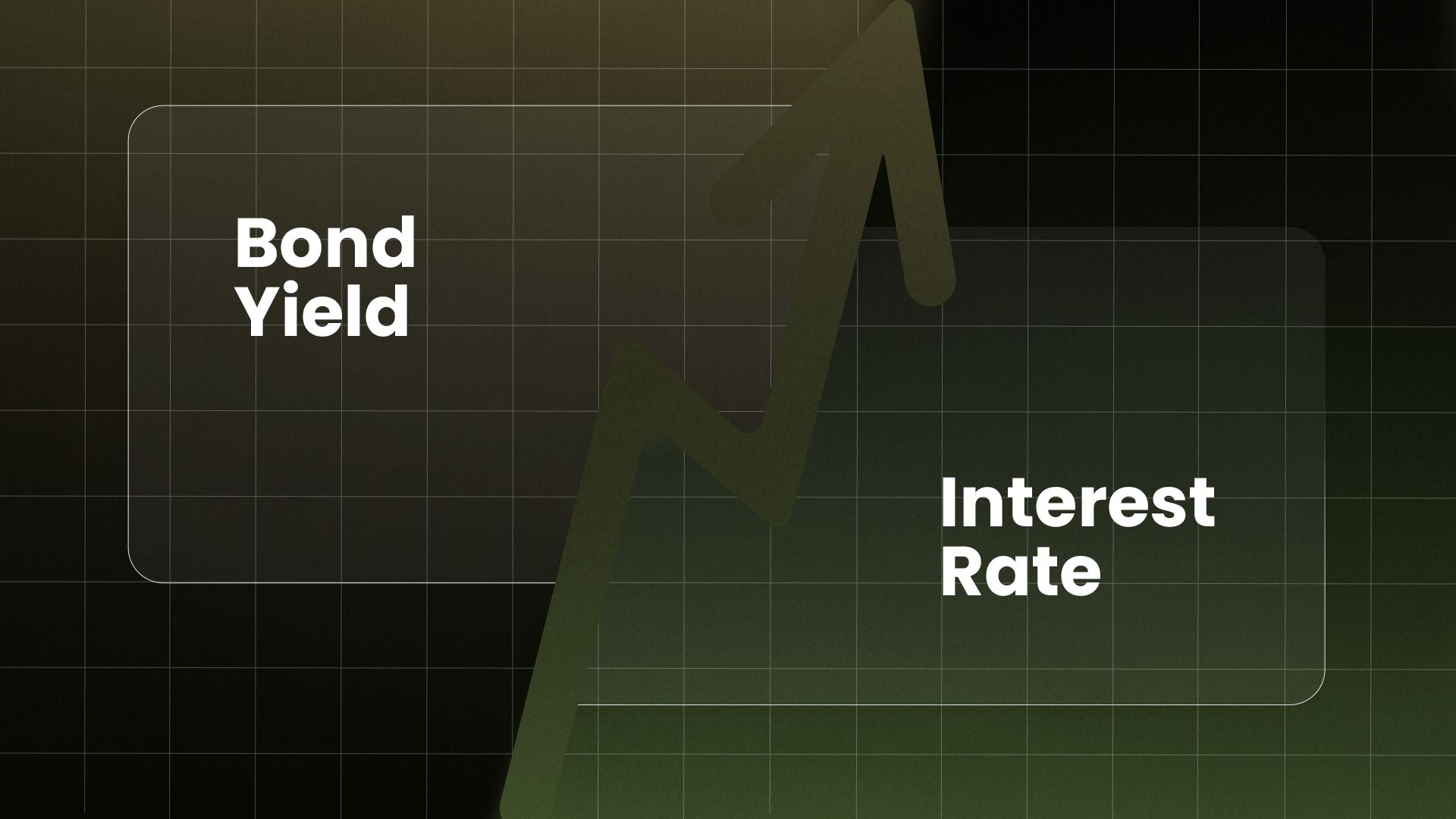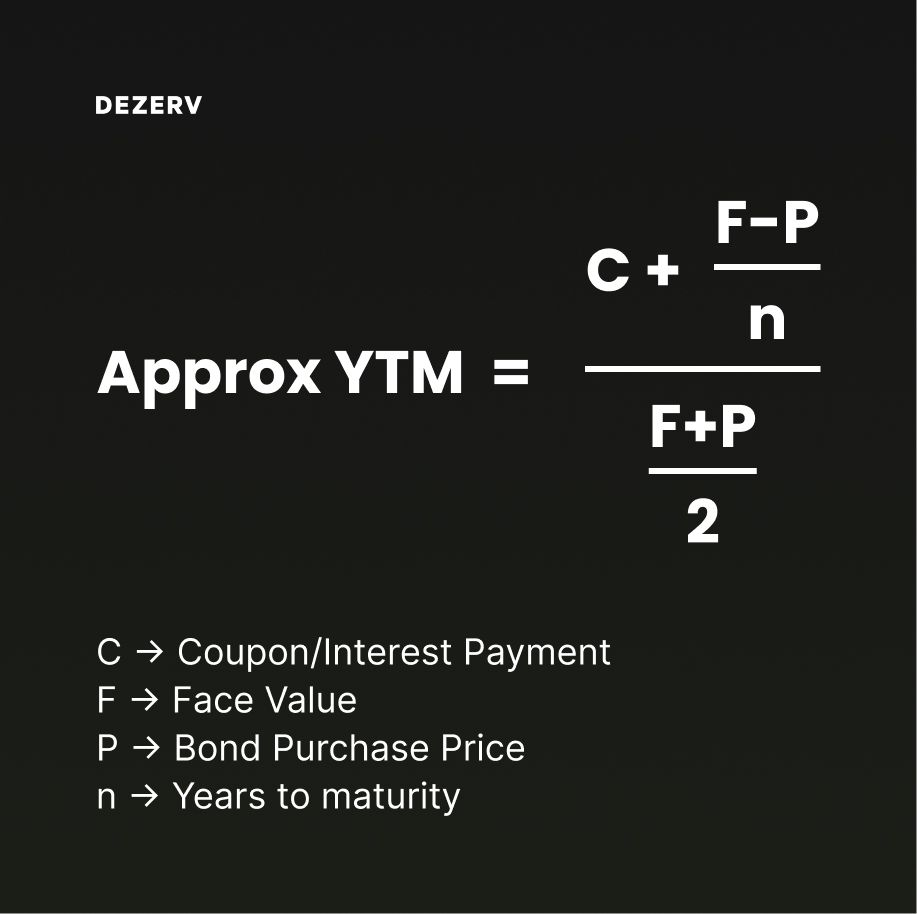# Bond yield vs Interest rateBond investing is picking up pace in India. After clocking a ~14% annual growth rate over the last 10 years, India’s bond market size is now a whopping \$1.8 trillion.

Govt platforms like RBI Retail Direct and many new-age companies have made it easy for investors to invest in Bonds and Debentures. Investors can explore and invest in bonds thanks to free and easily available educational content.

But there is one question bond investors struggle with the most:

## What is the Difference between Bond Yield and Interest Rate?

It is natural for the average investor to get confused when they see two numbers associated with bond returns. And it is not surprising. Bonds are complex instruments with several attributes.

Bond yield represents the annual return the bondholder earns, whereas interest rate determines the amount of interest the bondholder will receive.

We know this can be a bit confusing to understand.

So we wrote this article that simplifies bond yield and interest rate, and helps you understand the differences between the two.

## What is Bonds Interest Rate?

Before understanding the interest rate, let’s understand the face value of a bond since interest rate and face value are closely related.

The face value of a bond is what the investor receives from the bond issuer at maturity. It is generally a round number like Rs. 1,000 or Rs. 1 lakh. Face value is also called par value.

Many investors believe that the face value of the bond is the price of the bond; this is a common misconception. The bond price is generally close to the face value, but bonds mostly trade at a price that’s lower (at a discount) or higher (at a premium) than the face value of the bond. We discuss this in detail in the next section.

The face value and interest rate determine the quantum of interest payments bondholders will receive from the bond issuer.

Suppose a bond has an interest rate of 10% paid annually. In that case, the issuer will pay bondholders 10% of the face value as interest yearly.

In short, a bond's interest rate determines the quantum of interest payment the bondholders are entitled to receive.

The name of the bond specifies the interest rate of the bond. For example, the Government of India recently issued a sovereign green bond named 7.10% GOI SGrB 2028. The bond offers an interest rate of 7.10% and makes two interest payments every year.

## What is a bond yield?

Bond yield (YTM) indicates the annual return the investor will likely generate if he invests in the bond and holds it until maturity.

Bond yield calculation considers the bond price, the face value, the maturity date and the interest payment. The other 3 are fixed in most cases except for the bond price.

Further, YTM assumes that all the coupons received can be reinvested at the YTM rate. This assumption rarely holds, especially for bonds with longer tenures.

Hence, bond yield (YTM) is a dynamic number, unlike a bond’s interest rate, which is always fixed.

However, the bond yield (YTM) and interest rate are identical for investors when the purchase price (bond price) is the same as the face value of the bond.

It's logical because when the purchase price (bond price) is low, the bond yield (YTM) should be high.

Another way to look at this is that bond yield (YTM) and interest rate are the same when the purchase price (bond price) is equal to the selling price (face value). If the purchase price is less than the selling price, the returns (YTM) generated should naturally be higher.

### Yield to Maturity (YTM) formula

As we saw, the yield-to-maturity calculation requires 4 values: bond price, face value, the maturity date (details) and interest payment.